# A beam of light hits a plane mirror as shown below. Which letter indicates where the...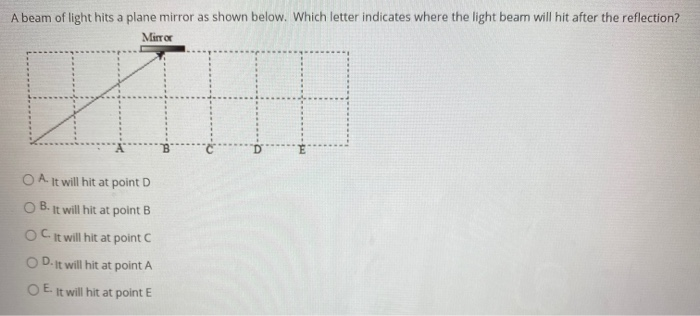A beam of light hits a plane mirror as shown below. Which letter indicates where the light beam will hit after the reflection? Mirror O A. It will hit at point D B. It will hit at point B OC. It will hit at point C D. It will hit at point A E. It will hit at point E

It will hit at point E.

Because, angle of incidence =angle of reflection

##### Add Answer of: A beam of light hits a plane mirror as shown below. Which letter indicates where the...
Similar Homework Help Questions
• ### A beam of light hits a plane mirror as shown below. Which letter indicates where the...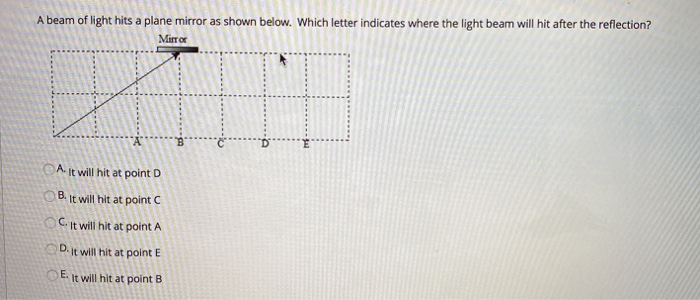A beam of light hits a plane mirror as shown below. Which letter indicates where the light beam will hit after the reflection? Mirror A It will hit at point D B. It will hit at point C C. It will hit at point A Dit will hit at point E E. It will hit at point B

• ### A beam of light hits a plane mirror as shown below. Which letter indicates where the...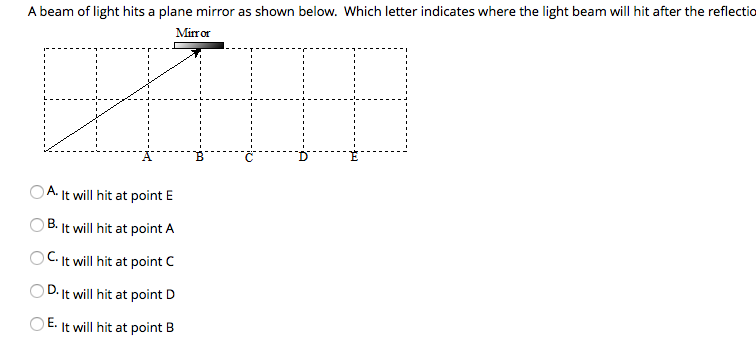A beam of light hits a plane mirror as shown below. Which letter indicates where the light beam will hit after the reflectio Mirror A. It will hit at point E B. It will hit at point A C. It will hit at point D. It will hit at point D It will hit at point B

• ### In each of the figures below, a light beam from hits a double slit plate with...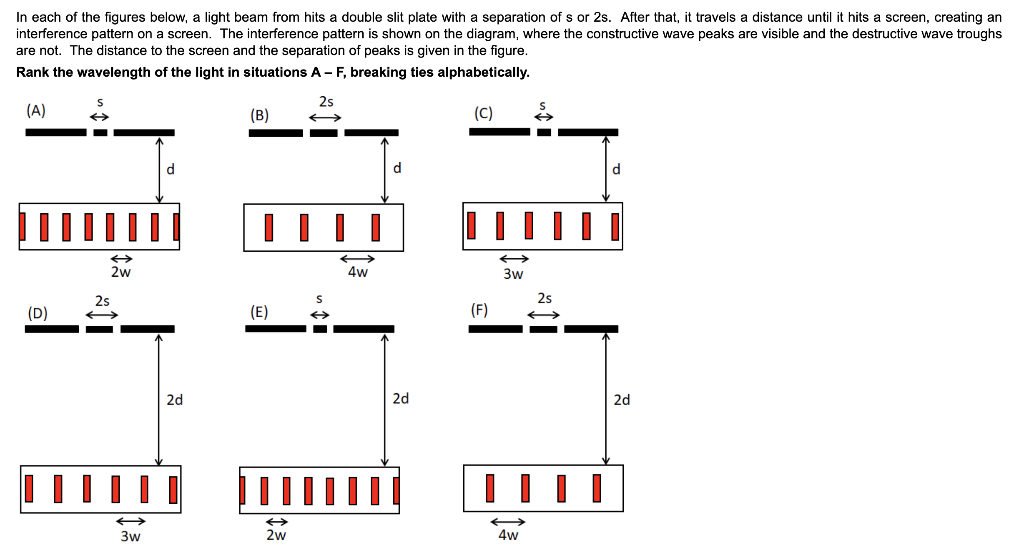In each of the figures below, a light beam from hits a double slit plate with a separation of s or 2s. After that, it travels a distance until it hits a screen, creating an interference pattern on a screen. The interference pattern is shown on the diagram, where the constructive wave peaks are visible and the destructive wave troughs are not. The distance to the screen and the separation of peaks is given in the figure. Rank the wavelength...

• ### 8) Two plane mirrors are arranged as shown below. A beam of light strikes the first...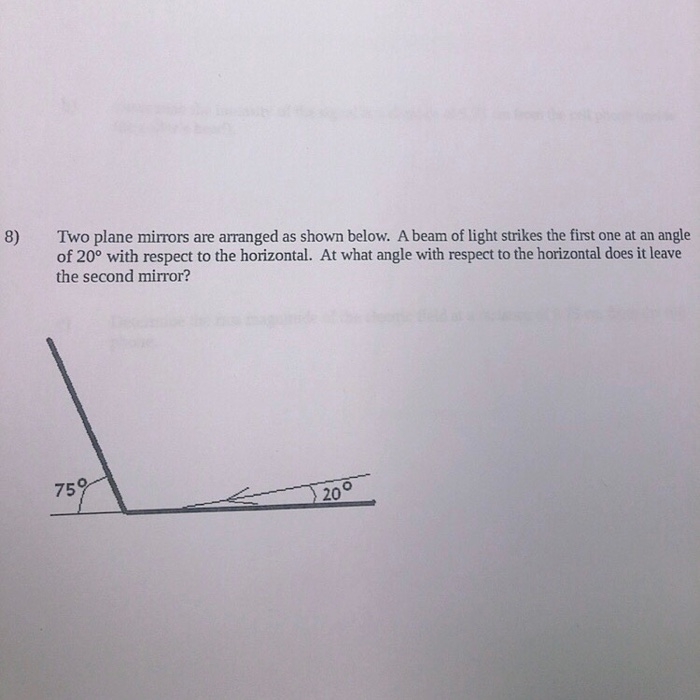8) Two plane mirrors are arranged as shown below. A beam of light strikes the first one at an angle of 20° with respect to the horizontal. At what angle with respect to the horizontal does it leave the second mirror? J 200

• ### Correct Answer: E (0.6m) 2. A laser beam is aimed at smooth, polished mirror laying fat...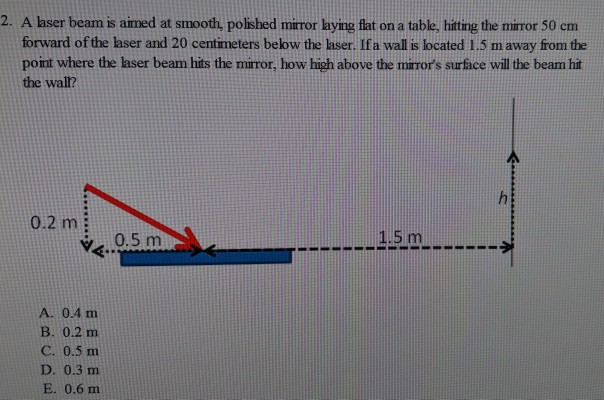Correct Answer: E (0.6m) 2. A laser beam is aimed at smooth, polished mirror laying fat on a table, hitting the miror 50 em forward of the laser and 20 centimeters below the laser. Ifa wall is located 1.5 m away from the point where the laser beam hits the mirror, how high above the mirror's surface will the beam hit the wall? 0.2 m 1.5 m v0.5 m A. 0.4 m B. 0.2 m C. 0.5 m D. 0.3...

• ### HOW MANY TIMES DOES THE LIGHT BEAM REFLECT FROM MIRROR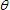Consider the light beam shown in the figure, where d =207 cm and = 12.5°.(a) How many times does the light beam reflectfrom the top mirror? times(b) How many times does the light beam reflect from the bottommirror? times

• ### help plsr LUCTUUR IU 1 pun An image is formed by a plane mirror. Where is...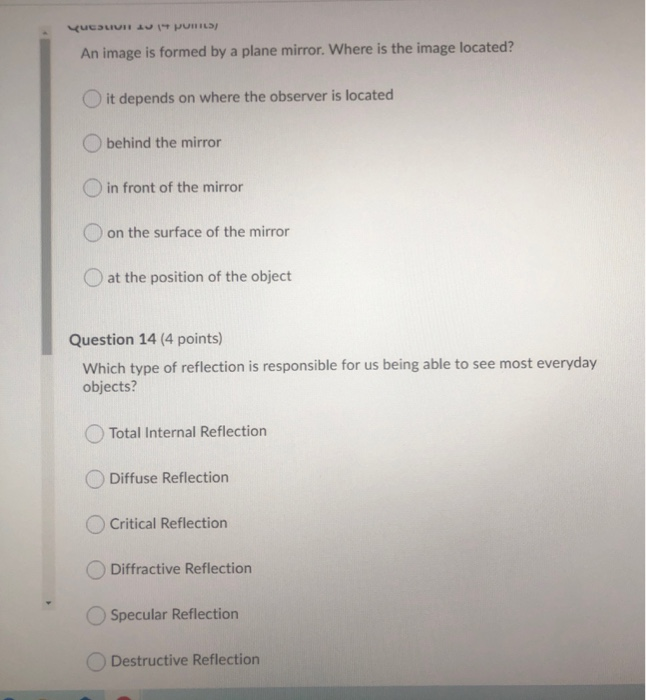help plsr LUCTUUR IU 1 pun An image is formed by a plane mirror. Where is the image located? O it depends on where the observer is located behind the mirror O in front of the mirror on the surface of the mirror at the position of the object Question 14 (4 points) Which type of reflection is responsible for us being able to see most everyday objects? Total Internal Reflection Diffuse Reflection Critical Reflection Diffractive Reflection Specular Reflection Destructive...

• ### In the mirror shown below, the angle of incident is 52º. 15) In the mirror shown...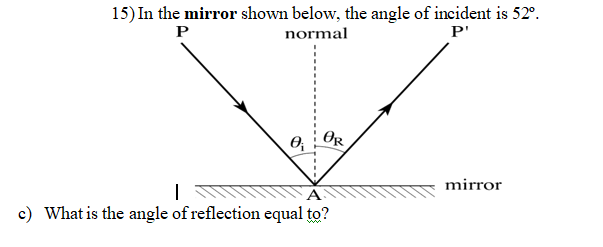In the mirror shown below, the angle of incident is 52º. 15) In the mirror shown below, the angle of incident is 52º. P normal P e OR | mirror c) What is the angle of reflection equal to?

• ### Light rays are produced by a light bulb and reflected on a plane mirror, before reaching...

Light rays are produced by a light bulb and reflected on a plane mirror, before reaching the eye of the observer Determine the location at which the observer sees the image of the light bulb. Approach. Note, the reflected rays are diverging. Therefore you need to extend the reflected rays backward. These lines should be drawn as dashed lines; this indicates that no light is really travelling this way ("virtual rays"), but this is where we think light is coming...

• ### beam of light!!!The two mirrors in Figure P22.6 meet at a right angle. The beam oflight in the vertical plane P strikes mirror 1 atθ = 37.5° as shown.Figure P22.6(a) Determine the distance the reflected lightbeam travels before striking mirror 2.1 m(b) In what direction does the light beam travel after beingreflected from mirror 2?2° (above the horizontal)

Free Homework App